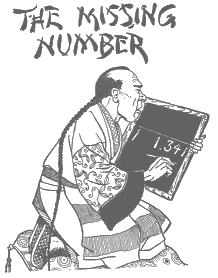Sam's missing number (1)Write down any two numbers, using the nine digits and zero. Make sure that each digit is used once and once only. For example, you can write 589641 and 2703. Add these two numbers. Here the sum total is 592344. Then erase one digit (any digit excluding 0) in this sum. So if I erase 2, the result is 59344. Enter this last result only. Then I'll tell you what digit you erased !

CLICK the picture

(1)   source : Mathematical puzzles by Sam Loyd